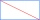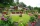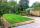# Perimeter + quadratic equation - math problems

#### Number of problems found: 31

• A isoscelesA isosceles triangle has an area of 168 cm2 and it's added height and base is 370 cm. What are the measurements of it's height and base?
• A rectangle 4A rectangle has area 300 and perimeter 80. what is the ratio of the length and width?
• Area and perimeter of rectangleThe content area of the rectangle is 3000 cm2, one dimension is 10 cm larger than the other. Determine the perimeter of the rectangle.
• Perimeter and diagonalThe perimeter of the rectangle is 82 m, the length of its diagonal is 29 m. Find the dimensions of the rectangle.
• Difference of legsIn a right triangle, the length of the hypotenuse is 65 m, and the difference of legs is 23 m. Calculate the perimeter of this triangle.
• Rectangle fieldThe field has a shape of a rectangle having a length of 119 m and a width of 19 m. , How many meters have to shorten its length and increase its width to maintain its area and circumference increased by 24 m?
• Square sideIf we enlarge the square side a = 5m, its area will increase by 10,25%. How many percent will the side of the square increase? How many percent will it increase the circumference of the square?
• Isosceles triangle 9Given an isosceles triangle ABC where AB= AC. The perimeter is 64cm, and the altitude is 24cm. Find the area of the isosceles triangle.
• Ratio of sidesCalculate the area of a circle with the same circumference as the circumference of the rectangle inscribed with a circle with a radius of r 9 cm so that its sides are in ratio 2 to 7.
• Rectangular garden 2A farmer bought 600 m of wire for the fence. He wants to use it to besiege a rectangular garden with a surface of 16875 m2. Calculate the size of the garden.
• Perimeter of RTFind the circumference of the rectangular triangle if the sum of its legs is 22.5 cm and its area is 62.5 cm2.
• AP RT triangleThe length of the sides of a right triangle forms an arithmetic progression, and the longer leg is 24 cm long. What are the perimeter and area?
• Rectangle - area, perimeterThe area of a rectangular field is equal to 300 square meters. Its perimeter is equal to 70 meters. Find the length and width of this rectangle.
• Right triangle eq2Find the lengths of the sides and the angles in the right triangle. Given area S = 210 and perimeter o = 70.
• RectangleThe rectangle has a perimeter 75 cm. Diagonal length is 32.5 cm. Determine the length of the sides.
• Right triangle eq2Hypotenuse of a right triangle is 9 cm longer than one leg and 8 cm longer than the second leg. Determine the circumference and area of a triangle.
• Rectangle poolFind dimensions of an open pool with a square bottom with a capacity of 32 m3 to have painted/bricked walls with the least amount of material.
• Rectangle diagonalsIt is given a rectangle with an area of 24 cm2 a circumference of 20 cm. The length of one side is 2 cm larger than the length of the second side. Calculate the length of the diagonal. Length and width are yet expressed in natural numbers.
• RT perimeterThe leg of the rectangular triangle is 7 cm shorter than the second leg and 8 cm shorter than the hypotenuse. Calculate the triangle circumference.
• GardenThe garden has a rectangular shape and has a circumference of 130 m and area 800.25 m2. Calculate the dimensions of the garden.

Do you have an exciting math question or word problem that you can't solve? Ask a question or post a math problem, and we can try to solve it.

We will send a solution to your e-mail address. Solved examples are also published here. Please enter the e-mail correctly and check whether you don't have a full mailbox.

Looking for help with calculating roots of a quadratic equation? Perimeter - math problems. Quadratic Equations Problems.